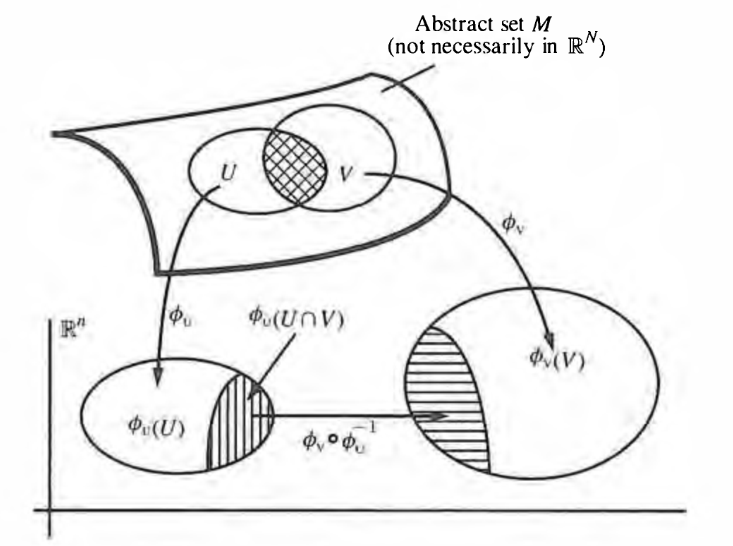Contents

# Contents

## IdeaA topological manifold $X$ of dimension $n \in \mathbb{N}$ is by definition a topological space that is locally homeomorphic to a Cartesian space $\mathbb{R}^n$. A choice of such morphism

$\phi : \mathbb{R}^n \stackrel{\simeq}{\to} X|_{im(\phi)} \hookrightarrow X$

is a coordinate system or coordinate chart or just chart on the image of $\phi$.

This generalises to other sorts of manifolds.

An atlas is the collection of coordinate charts defining a manifold structure.

graphics grabbed from Frankel

Last revised on August 1, 2018 at 12:04:18. See the history of this page for a list of all contributions to it.